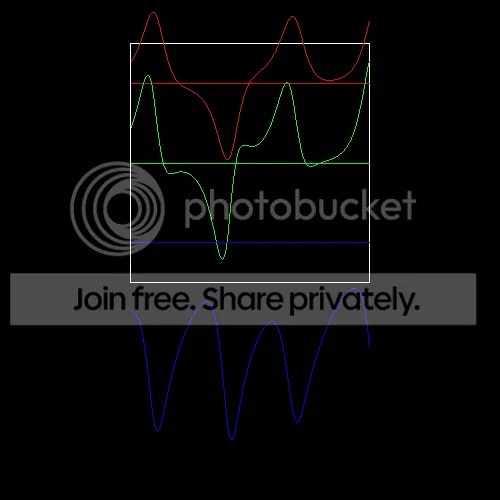Bizarre Love Triangle Explained

Earlier I wrote about issues I have with Tuples and Functions. It was kind of long, rambling and tangential, so I decided to write about some concrete examples of what I’m talking about.

My first example pertains to the FunctionN implementations. For the linear transformation drawer, I tried writing a DSL with some sort of function application syntax that maps to underlying Scala methods (e.g. a Parser for “functionCall(arg0, arg1, … argX)”). I would like to simply write one method that takes any function and gives back a Parser for that function:

```def makeParser(functionX:SomeFunctionType):Parser[FunctionReturnType] = { ... }

val absParser = makeParser(math.abs _)
val powParser = makeParser(math.pow _)
```

What do I put in for SomeFunctionType? Nothing makes sense. Instead, I have to write makeParser1Arg, makeParser2Arg, etc. Code repetition should be avoided – but in this case, there’s no way around it. Even the Scala core library “solves” this problem through code repetition (that is, Scala has a TON of copypasta in FunctionN and TupleN).

As a second example, I wrote a simple grapher for the Lorenz Attractor that plotted each individual coordinate over time. I wanted to show each coordinate in a different color and also laid out below each other:So I wrote a method that looked like this:

```def draw(color:Int, y:Float, list:Seq[Float]) = {
translate(0, y);
stroke(color);
//handle draw...
}
```

I have a Seq[(Float, Float, Float)] representing the attractor’s states as it progresses through time. I can use unzip3 to get a (Seq[Float], Seq[Float], Seq[Float]) holding each individual coordinate as it progresses through time. Now, I want to call draw three times, passing each Seq as the third argument. I could hard-code it like so:

```//the original seq of the attractor's states
val states:Seq[(Float, Float, Float)] = ...

//Three Seqs of individual coordinates
val coords = states.unzip3;

draw(color(255, 0, 0), 0, coords._1);
draw(color(0, 255, 0), height/3f, coords._2);
draw(color(0, 0, 255), height*2/3f, coords._3);
```

The code repetition is pretty blatant. What if I wanted to draw 10 different lines? What about 100? Instead of explicitly calling draw with different values, I’d like to create two other containers – be them lists, tuples, whatever – holding the corresponding colors and y coordinates to be passed to the draw method.

```val colors = List(color(255, 0, 0), color(0, 255, 0), color(0, 0, 255));
val ys     = List(0f, height/3f, height*2/3f);
```

FunctionN’s have a .tupled method to let you pass a single TupleN to the function, letting you treat the arguments to pass to a Function as one object. All I have to do now is combine the three coords, colors, and ys Seqs into one Seq of Tuple3s, with each Tuple3 holding the corresponding arguments to pass to draw.

```//combine colors, ys, and coords somehow
val args:Seq[(Int, Float, Seq[Float])] = ...

args foreach (draw _).tupled //pass each set of args to the draw method
```

How do I do it? The answer is: very ugily (like, ugly-ly?). At the very least, you have to convert the coords from a Tuple3 of Seq[Float]s into a Seq of Seq[Float]s. There is no pretty way to do this. You can explicitly code it:

```//ewwwww
val coordsList = Seq(coords._1, coords._2, coords._3);
```

Or you can use the productIterator method of Tuple3, and then cast to an iterator of Seq[Float]s:

```//oh my jesus
val coordsList = coords.productIterator.asInstanceOf[Iterator[Seq[Float]]];
```

The first option isn’t extensible (if you want to plot a 5-dimensional attractor you’ll need to go back and explicitly change the code); the second option isn’t type-safe (if coords isn’t actually ALL Seq[Float]s, this won’t even exception until we try to actually explicitly grab one of the elements, at god knows what place).

But lets say we’ve gotten beyond that. Now we want to create the args sequence. We basically want the inverse of unzip3, taking in three seq arguments (one of them can be the caller) and outputting one Seq of Tuple3s. Seq has a “zip” method that does very, very close to what we want; Seq.zip only takes one argument and outputs a Seq of Tuple2s. But it’s not good enough. You can’t chain a zip with another zip, because that still only creates a Tuple2.

```//Seq[(Int, Float)]
val args2 = colors.zip(ys)

//Seq[((Int, Float), Seq[Float])]
val almost = args2.zip(coordsList)
```

Here are some solutions I’ve ended up with:

```//iterate through the index
val args = for(i &lt;- 0 until colors.length) yield (colors(i), ys(i), coordsList(i))

//same thing only slower! wooooo!
val args = colors.zipWithIndex.map { case (c, i) =&gt; (c, ys(i), coordsList(i)) }

//actually not terrible
val args = almost.map{ case ((a, b), c) =&gt; (a, b, c) }
```